Trang chủ / Toán Kangaroo / Lớp 7-8

1. The product of two numbers is 36 and their sum is 37. What is their difference?

2. What is the result of: 2014 × 2014 ÷ 2014 – 2014?

3. Wanda had several square pieces of paper, each piece of an area of 4. She cut them into squares and right-angled triangles in the manner shown in the first diagram.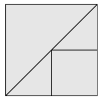She then took some of the pieces and made the bird shown in the second diagram.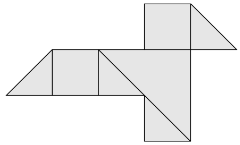What is the area of the bird?

4. The number 100 can be written as a sum of powers of 2. What is the least number of powers of two, the sum of which equals 100?

5. The area of the rectangle ABCD is 10. The points M and N are the midpoints of the sides AD and BC . What is the area of the quadrilateral MBND ?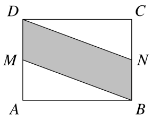6. A bucket was half full. A cleaner added 2 litres to the bucket. The bucket was then three-quarters full. What is the capacity of the bucket?

7. George built the shape shown using seven unit cubes . How many such cubes does he have to add to make a cube with edges of length 3?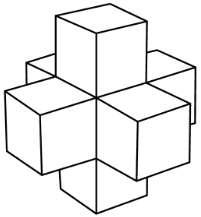8. What is the positive number whose reciprocal is equal to its quadruple?

9. Which of the following calculations gives the large st result?

10. The necklace in the picture contains dark grey bead s and white beads.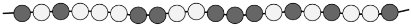Arno takes one bead after another from the necklace . He always takes a bead from either one of the ends. He stops as soon as he has taken the fifth grey bead. What is the largest number of white beads that Arno can take?

11. Part B: Each correct answer is worth 4 points

Jack has a piano lesson twice a week and Hannah has a piano lesson every other week. In a given term, Jack has 15 more lessons than Hannah. How man y weeks long is their term?

12. In the diagram, the area of each circle is 1cm2 . The area common to two overlapping circles is 1/8  cm2 . What is the area of the region covered by the five circles?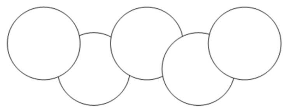13. The diagram shows the triangle ABC in which BH is a perpendicular height and AD is the angle bisector at A . The obtuse angle between BH and AD is four times the angle DAB (see the diagram). What is the measure of the angle CAB ?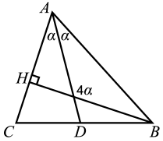14. Six boys share an apartment with two bathrooms, which they use every morning beginning at 7:00am. There is never more than one person in either bathroom at any one time. They spend 8, 10, 12, 17, 21 and 22 minutes at a stretch in the bathroom respectively. What is the earliest time that they can finish using the bathrooms?

15. Nine lamps are arranged in a square. Any lamp can b e on or off. If you push on a lamp, the status of the lamp and that of each lamp in the same row and the same column changes (if it is on, it goes off, and vice versa). In the beginning all lights a re on. At least how many pushes are needed to turn all the lamps off?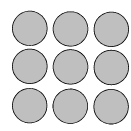16. Five identical rectangles are placed inside a square with side 24 cm, as shown in the diagram. What is the area of one rectangle?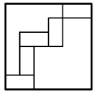17. A rectangle has sides of length 6 cm and 11 cm. One long side is selected. The bisectors of the angles at either end of that side are drawn. These bisectors divide the other long side into three parts. What are the lengths of these parts?

18. The neighbours of a two-digit number are a prime number and a perfect square (a square of a whole number). How many two-digit numbers are there with this property?

19. The average of two positive numbers is 30% less than one of them, and X% more than the second one. What is the value of X?

20. Quadrilateral ABCD has right angles only at vertices A and D. The numbers show the areas of two of the triangles. What is the area of ABCD?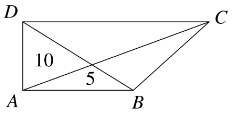21. Part C: Each correct answer is worth 5 points

An old scale is not working properly. If something is lighter than 1000 grams, the scale shows the correct weight. However, if an object's weight is greater than or equal to 1000 grams, the scale can show any number above 1000 grams. We have 5 weightsA grams, B grams, C grams, D grams and E grams each under 1000 grams. When they are weighed in pairs, the scale shows the following: B + D = 1200, C + E = 2100, B + E = 800, B + C = 900, A + E = 700. Which of the weights is the heaviest?

22. Liz and Mary competed in solving problems. Each of them received the same list of 100 problems. For any problem, the girl who solved the problem first got 4 points, whereas the girl who solved the problem second got 1 point. Liz solved 60 problems and Mary also solved 60 problems. Together, they got 312 points. How many problems were solved by both of them?

23. In the picture below, there are four identical cubes seen from different perspectives.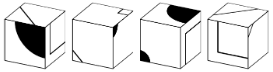They are arranged so that a big black disk appears on one face, as shown in the picture.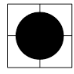What can be seen on the opposite face?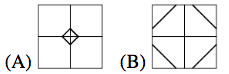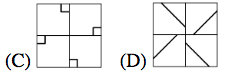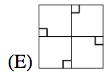24. A group of 25 people consists of knights, serfs and damsels. Each knight always tells the truth, each serf always lies, and each damsel alternates between telling the truth and lying. Thus, if a damsel answers truthfully to a question, she will always lie on the next question, and tell the truth again on the question after that.
When each of the 25 persons was asked: "Are you a knight?", 17 of them said "Yes".
When each of them was then asked: "Are you a damsel?", 12 of them said "Yes".
When each of them was then asked: "Are you a serf?", 8 of them said "Yes".
How many knights are in the group?

25. Several different positive integers are written on the board. Exactly two of them are divisible by 2 and exactly 13 of them are divisible by 13. Let M be the greatest of these numbers. What is the smallest possible value of M?

26. David rode his bicycle from Edinburgh to his farm. He was going to arrive at 3:00 pm, but he spent 2/3 of the planned time covering 3/4 of the distance. After that, he rode more slowly and arrived exactly as expected. What is the ratio of the speed for the first part of the journey to the speed for the second part?

27. Captain Sparrow and his pirate crew dug up several gold coins. They are dividing the coins amongst themselves so that each person gets the same number of coins. If there were four fewer pirates, then each person would get 10 more coins. However, if there were 50 fewer coins, then each person would get 5 fewer coins. How many coins did they dig up?

28. On a pond there are 16 water lily leaves in a 4 by 4 pattern as shown. A frog sits on a leaf in one of the corners. It then jumps from one leaf to another either horizontally or vertically. The frog always jumps over at least one leaf and never lands on the same leaf twice. What is the greatest number of leaves (including the one it sits on) that the frog can reach?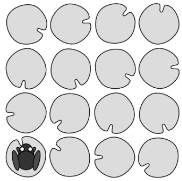29. Ten children are lined up from tall to small. They have to be lined up from small to tall. This will be achieved by a number of interchanges of two children next to each other. How many interchanges are needed?

30. The dome of Canada’s largest church, the Oratory St. Joseph in Montreal, is surrounded by a decorative ring-shaped motif composed of congruent regular pentagons, congruent isosceles triangles, and congruent isosceles trapezoids. The diagram shows a fragment of this motif.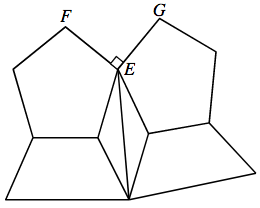If it is known that the angle FEG is right, what is the measure of the acute angles of the trapezoids?